1587459600

# Python Tutorial – A Comprehensive Guide to Learn Python Programming

Python is a high-level, object-oriented, interpreted programming language, which has garnered worldwide attention. Stack Overflow found out that 38.8% of its users mainly use Python for their projects. According to the website’s survey, Python’s popularity surpassed that of C# in 2018 – just like it surpassed PHP in 2017 and right now python users have surpassed the Java users with 8.2 million users as of 2020. In this Python Tutorial, I will be discussing the following topics:

• Introduction To Python
• What is Python?
• Python Interpreter
• Python Installation
• Python Installation For Windows
• Python Installation For Linux
• Python Installation For Mac
• Python IDE
• Which Python IDE?
• PyCharm
• Jupyter Notebook
• Why Learn Python?
• Python Applications
• Python Basics
• Python Keywords
• Python Variables
• Global Variables In Python
• Data Types In Python
• Python Strings
• Python Strings Format
• Python Strings Methods
• Python Strings Operations
• Numbers
• Python Integers
• Python Float
• Python Boolean
• Python Lists
• Python Lists Remove
• Python List Remove Duplicates
• Python Tuples
• Python Sets
• Python Dictionary
• Dictionary Methods
• Operators In Python
• Conditional Statements
• If,Else,Elif Statement In Python
• Conditional Statement Example
• Loops In Python
• For Loop In Python
• While Loop In Python
• Nested Loops In Python
• Python Functions
• File I/O In Python
• Python Modules
• Built-in Modules In Python
• OS
• Math
• Random
• Datetime
• Python OOPs
• Python Classes
• Inheritance In Python

## **Introduction To Python **

Python in simple words is a High-Level Dynamic Programming Language which is interpreted. Guido Van Rossum, the father of Python had simple goals in mind when he was developing it, easy looking code, readable and open source. Python is ranked as the 3rd most prominent language followed by JavaScript and Java in a survey held in 2018 by Stack Overflow which serves proof to it being the most growing language.

### What is Python?

Python is currently my favorite and most preferred language to work on because of its simplicity, powerful libraries, and readability. You may be an old school coder or may be completely new to programming, Python is the best way to get started!

Python provides features listed below :

• ``````Simplicity: Think less of the syntax of the language and more of the code.
``````
• ``````Open Source: A powerful language and it is free for everyone to use and alter as needed.
``````
• ``````Portability: Python code can be shared and it would work the same way it was intended to. Seamless and hassle-free.
``````
• ``````Being Embeddable & Extensible: Python can have snippets of other languages inside it to perform certain functions.
``````
• ``````Being Interpreted: The worries of large memory tasks and other heavy CPU tasks are taken care of by Python itself leaving you to worry only about coding.
``````
• ``````Huge amount of libraries: Data Science? Python has you covered. Web Development? Python still has you covered. Always.
``````
• ``````Object Orientation: Objects help breaking-down complex real-life problems into such that they can be coded and solved to obtain solutions.
``````

To sum it up, Python has a simple syntax, is readable, and has great community support.

## Python Interpreter

An interpreter is a computer program that directly executes, i.e. performs, instructions written in a programming or scripting language, without requiring them previously to have been compiled into a machine language program. So unlike Java, Python uses an interpreter.

The interpreter is a program that we need to run our python code or scripts. It basically provides an interface between the code and the computer hardware to get the results of the code.

No matter in which programming language the code is written, it goes through an interpreter when it comes to python. For example pypy is used to execute the code written in python.

## Python Installation

I will be installing Python in Windows 10 OS. You can try installing Python in Linux, Mac etc. If you face any issue mention it in the comments section.

Following are the steps to install Python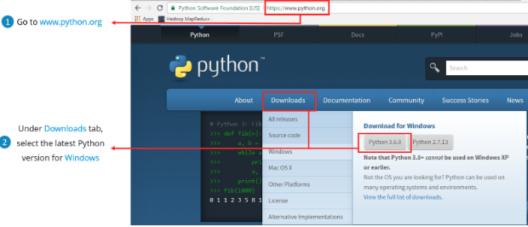## Python Installation For Windows

Python Installation For Windows

1. ``````Open a browser window and navigate to the [Download page for Windows](https://www.python.org/downloads/windows/ "") at [python.org](https://www.python.org/ "").
``````
2. ``````Underneath the heading at the top that says Python Releases for Windows, click on the link for the Latest Python 3 Release – Python 3.x.x. (As of this writing, the latest version is Python 3.7.2.)
``````
3. ``````Scroll to the bottom and select either Windows x86-64 executable installer for 64-bit or Windows x86 executable installer for 32-bit.
``````

But do we pick 32-bit or the 64-bit installer?

For Windows, you can choose either the 32-bit or 64-bit installer. Here’s what the difference between the two comes down to:

• ``````If your system has a 32-bit processor, then you should choose the 32-bit installer.
``````
• ``````On a 64-bit system, either installer will actually work for most purposes. The 32-bit version will generally use less memory, but the 64-bit version performs better for applications with intensive computation.
``````
• ``````If you’re unsure which version to pick, go with the 64-bit version.
``````

Open the installer and click on “Run”.

#### Python Installation For Linux

Use the Graphical linux installation to install python on linux. Follow the steps below:

• ``````Open the Ubuntu Software Center folder.
``````
• ``````Select Developer Tools (or Development) from the All Software drop-down list box.
``````
• ``````Double-click the Python 3.3.4 entry.
``````
• ``````Click Install.
``````
• ``````Close the Ubuntu Software Center folder.
``````

#### Python Installation For Mac

Before installing Python, you’ll need to install GCC. GCC can be obtained by downloading Xcode, the smaller Command Line Tools or the even smaller OSX-GCC-Installer package.

While OS X comes with a large number of Unix utilities, those familiar with Linux systems will notice one key component missing: a package manager. Homebrew fills this void.

Once you’ve installed Homebrew, insert the Homebrew directory at the top of your PATH environment variable. You can do this by adding the following line at the bottom of your ~/.profile file

``````export PATH="/usr/local/opt/python/libexec/bin:\$PATH"
``````

Now, we can install Python 3:

``````\$brew install python
``````

### Python IDE

IDE typically provides code editor, compiler/ interpreter and debugger in one GUI (Graphical User Interface). It encapsulates the entire process of code creation, compilation and testing which increases the productivity of developers.

A developer working with an IDE starts with a model, which the IDE translates into suitable code. The IDE then debugs and tests the model-driven code, with a high level of automation. Once the build is successful and properly tested, it can be deployed for further testing through the IDE or other tools outside of the IDE.

#### Which Python IDE?

Always keep the following points in mind while choosing the best IDE for Python:

• ``````Level of expertise (beginner, professional) of the programmer
``````
• ``````The type of industry or sector where Python is being used
``````
• ``````Ability to buy commercial versions or stick to the free ones
``````
• ``````Kind of software being developed
``````
• ``````Need to integrate with other languages
``````

Once these points are decided upon, the programmer can easily select among the IDEs based on the given features.

#### PyCharm

Developed by Czech company JetBrains, PyCharm is an IDE specific to Python. PyCharm is a cross-platform IDE. Therefore, users may download any of the Windows, Mac or Linux versions depending on their requirements. Honestly, PyCharm is considered to be one of the best IDE for Python and justifiably the most widely used.

• ``````Specialized project views allowing quick switching between files
``````
• ``````Facilitates Web Development along with Django, Flask, and web2py
``````
• ``````PyCharm is equipped with more than 1000 plug-ins, so programmers can write their own plug-ins to extend its features
``````

It provides two versions for download, the Community version which is free and the paid Professional version. Programmers can download the corresponding versions as per their requirements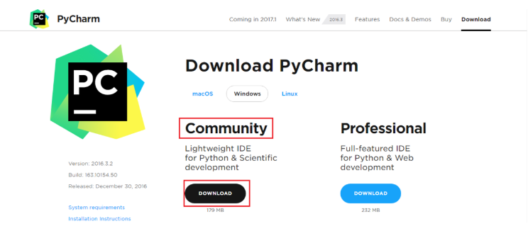Here, the community version is free, but for the professional version, you need to buy the license. I will be working on the PyCharm community version.

#### PyCharm Community Edition

let us dive right into Pycharm now and I will walk you through the interface.

Let us begin by creating a new project. PyCharm greets you with the following image as soon as you open it: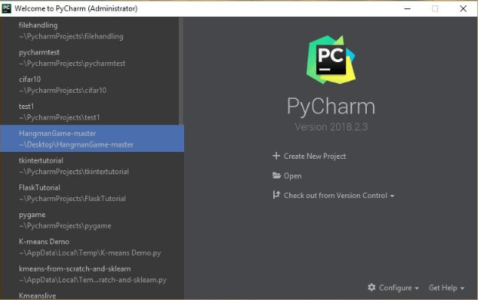Here, on the left, you can check out all of the recent projects that I have been working with. But if this is your first time using PyCharm, then the column on the left will not contain anything.

Creating a new project is as easy as clicking on the Create New Project tab and going from there.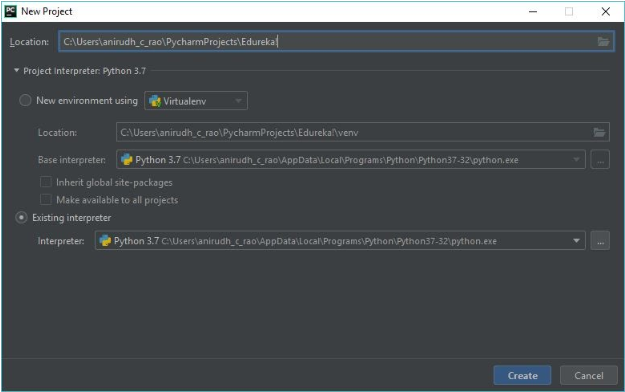This image basically helps us set up our Python Interpreter. However, this might be blank if you do not have Python installed on your system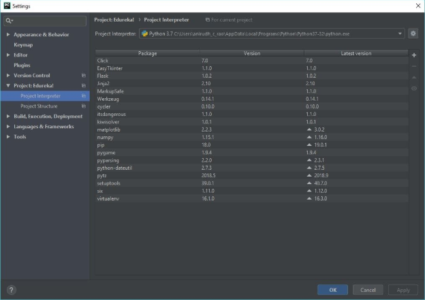Clicking on the little gear icon on the right will bring up the following page: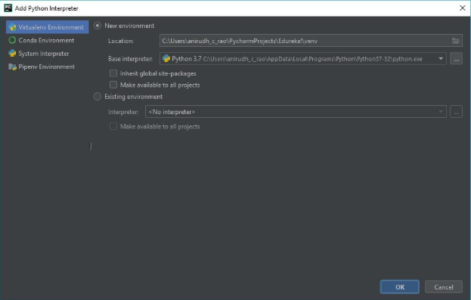Here, you can make use of the System Interpreter if you have not installed Python separately. Do check the version of the Python installation that is present so it meets your requirements.

#### Jupyter Notebook

Jupyter Notebooks are a powerful way to write and iterate on your Python code for data analysis. Jupyter Notebook is built off of IPython and the Kernel runs the computations and communicates with the Jupyter Notebook front-end interface.

Jupyter Notebook is an open-source web application that allows you to create and share documents that contain live code, equations, visualizations and narrative text. It is used for data cleaning and transformation, numerical simulation, statistical modeling, data visualization, machine learning, and much more.

### Why Learn Python?

Python’s syntax is very easy to understand. The lines of code required for a task is less compared to other languages. Let me give you an example – If I have to print “Welcome To Edureka!” all I have to type:

``````print(“Welcome To Morioh!”)
``````

Let’s look at some cool features of Python:

1. ``````Simple and easy to learn
``````
2. ``````Free and Open Source
``````
3. ``````Portable
``````
4. ``````Supports different programming paradigm
``````
5. ``````Extensible
``````

If you are wondering where you can use Python (Python Application), let me tell you that is where Python stands out.

### Python Applications

1. ``````Artificial Intelligence
``````
2. ``````Desktop Application
``````
3. ``````Automation
``````
4. ``````Web Development
``````
5. ``````Data Wrangling, Exploration And Visualization
``````

Let us now start coding in Python.

## Python Basics

The basic concepts in any programming language are the foundation of any programmer, We will start with the most basic concept in python.

### Python Keywords

Keywords are nothing but special names that are already present in python. We can use these keywords for specific functionality while writing a python program.

Following is the list of all the keywords that we have in python: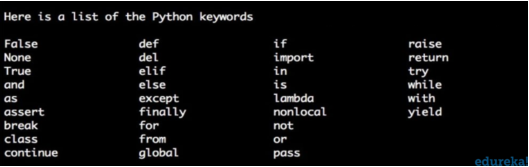Syntax and Usage

``````import keyword
keyword.kwlist
#this will get you the list of all keywords in python.
keyword.iskeyword('try')
#this will return true, if the mentioned name is a keyword.
``````

Identifiers are user defined names that we use to represent variables, classes, functions, modules etc.

Syntax and Usage

``````name = 'edureka'
my_identifier = name
``````

programmer-coherent statements, that describe what a block of code means. They get very useful when you are writing large codes. It’s practically inhuman to remember the names of every variable when you have a hundred-page program or so. Therefore, making use of comments will make it very easy for you, or someone else to read as well as modify the code.

Syntax

``````#this is a single line comment
#this is a multi
#line comment
"""this is a docstring
comment
i hope it is clear
"""
``````

A block comment in python is written with the same indentation as the code, it is used to explain the code. A block comment looks something like the one written in the example below.

``````# this is a print statement
print(“hello world”)
``````

### Python Variables

Variables are nothing but reserved memory locations to store values. This means that when you create a variable you reserve some space in memory.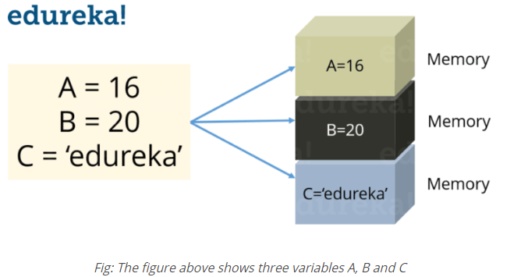In Python you don’t need to declare variables before using it, unlike other languages like Java, C etc.

#### Assigning values to a variable

Python variables do not need explicit declaration to reserve memory space. The declaration happens automatically when you assign a value to a variable. The equal sign (=) is used to assign values to variables. Consider the below example:

Syntax

``````S = 10
print(S)
``````

This will assign value ‘10’ to the variable ‘S’ and will print it. Try it yourself.

#### Python Global Variable

In python, GLOBAL Keyword can be used to access/modify the variables out of the current scope.

Syntax

``````A = 10
Def function():
global a
A += 10
print(A)
print(A)
function()
``````

### Data Types In Python

Python supports various data types, these data types defines the operations possible on the variables and the storage method. Below is the list of standard data types available in Python: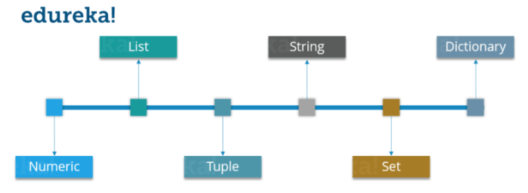Let’s discuss each of these in detail. In this Python tutorial, we’ll start with ‘STRINGS’ data type.

#### Python Strings

Strings are among the most popular data types in Python. We can create them simply by enclosing characters in quotes. Python treats single and double quotes in exactly the same fashion. Consider the example below:

Syntax

``````S= "Welcome To Morioh!"
D = 'Morioh!'
``````

#### Python String Methods

Some of the string methods used in python are written below:

1. ``````strip()
``````
2. ``````Count()
``````
3. ``````split()
``````
4. ``````translate()
``````
5. ``````index()
``````
6. ``````format()
``````
7. ``````find()
``````
8. ``````center()
``````
9. ``````join()
``````

#### Python String Operations#### Formatting A String In Python

Formatting a string means to allocate the string dynamically wherever you want.

Strings in Python can be formatted with the use of format() method which is very versatile and powerful tool for formatting of Strings. Format method in String contains curly braces {} as placeholders which can hold arguments according to position or keyword to specify the order.

Example

``````String1 = "{1} {0} {2}".format('Hello', 'to', 'Batman')
print("nPositional order: ")
print(String1)
``````

**Output:

``````Positional order:
to Hello Batman
``````

#### Python Numbers

Just as expected Numeric data types store numeric values. They are immutable data types, this means that you cannot change its value. Python supports three different Numeric data types:

#### Python Integer

It holds all the integer values i.e. all the positive and negative whole numbers, example – 10.

#### Python Float

It holds the real numbers and are represented by decimal and sometimes even scientific notations with E or e indicating the power of 10 (2.5e2 = 2.5 x 102 = 250), example – 10.24.

#### Python Complex

These are of the form a + bj, where a and b are floats and J represents the square root of -1 (which is an imaginary number), example – 10+6j.

#### Python Boolean

These are the decisive data types, they only return categorical value, i.e true or false.

Now you can even perform type conversion. For example, you can convert the integer value to a float value and vice-versa. Consider the example below:

Syntax And Usage

``````A = 10
# Convert it into float type
B = float(A)
print(B)
``````

The code above will convert an integer value to a float type. Similarly you can convert a float value to integer type:

``````A = 10.76
# Convert it into float type
B = int(A)
print(B)
``````

#### Python Lists

You can consider the Lists as Arrays in C, but in List you can store elements of different types, but in Array all the elements should of the same type.

List is the most versatile datatype available in Python which can be written as a list of comma-separated values (items) between square brackets. Consider the example below:

Syntax

``````subjects = ['physics', 'chemistry','biology']
print(subjects)
``````

Output:

`['physics, 'chemistry','biology']`

Notice that the Subjects List contains both words as well as numbers. Now, let’s perform some operations on our Subjects List.

Let’s look at few operations that you can perform with Lists:#### Python Lists Remove

``````a = [1,2,3,4,11,5]

a.remove(11)
#this will remove 11 from the list.
``````

#### Python Lists Remove Duplicates

Follow the steps below to remove duplicates from a list

1. ``````Create a dictionary with the same values from the list
``````
2. ``````Convert it into a list
``````
3. ``````Print the converted list. You will get a list with duplicates removed.
``````
``````Mylist = [a,b,c,b,c,a,c,a]
b = list(dict.fromkeys(Mylist))
print(b)
``````

#### Python Tuples

A Tuple is a sequence of immutable Python objects. Tuples are sequences, just like Lists. The differences between tuples and lists are:

• ``````Tuples cannot be changed unlike lists
``````
• ``````Tuples use parentheses, whereas lists use square brackets. Consider the example below:
``````

Syntax

``````chelsea = ('hazard', 'terry', 'lampard')
``````

Now you must be thinking why Tuples when we have Lists?

So the simple answer would be, Tuples are faster than Lists. If you’re defining a constant set of values that you just want to iterate, then use Tuple instead of a List.

Guys, all Tuple operations are similar to Lists, but you cannot update, delete or add an element to a Tuple.

Now, stop being lazy and don’t expect me to show all those operations, try it yourself.

#### Python Sets

A Set is an unordered collection of items. Every element is unique.

A Set is created by placing all the items (elements) inside curly braces {}, separated by a comma. Consider the example below:

Syntax

``````set_name = {1,2,3,4,5}
``````

In Sets, every element has to be unique. Try printing the below code:

``````set_2 = {1,2,3,3,4,5}
``````

Here 3 is repeated twice, but it will print it only once.

Let’s look at some Set operations:

#### Union

Union of A and B is a set of all the elements from both sets. Union is performed using | operator. Consider the below example:

``````A = {1, 2, 3, 4}
B = {3, 4, 5, 6}
print ( A | B)
``````

Output = {1, 2, 3, 4, 5, 6}

#### Intersection

Intersection of A and B is a set of elements that are common in both sets. Intersection is performed using & operator. Consider the example below:

``````A = {1, 2, 3, 4}
B = {3, 4, 5, 6}
print ( A & B )
``````

Output: `{3,4}`

#### Difference

Difference of A and B (A – B) is a set of elements that are only in A but not in B. Similarly, B – A is a set of element in B but not in A. Consider the example below:

``````A = {1, 2, 3, 4, 5}
B = {4, 5, 6, 7, 8}
print(A - B)
``````

Output: `{1,2,3}`

#### Python Dictionary

Now let me explain you Dictionaries with an example.

I am guessing you guys know about Aadhaar Card. For those of you who don’t know what it is, it is nothing but a unique ID which has been given to all Indian citizen. So for every Aadhaar number, there is a name and few other details attached.

Now you can consider the Aadhaar number as a ‘Key’ and the person’s detail as the ‘Value’ attached to that Key.

Dictionaries contains these ‘Key Value’ pairs enclosed within curly braces and Keys and values are separated with ‘:’. Consider the below example:

Syntax

``````dictionary_name = {key: value, key2: value2}
``````

You know the drill, now comes various Dictionary operations.

#### Access elements from a dictionary:

``````Dict = {'Name' : 'Saurabh', 'Age' : 23}
print(Dict['Name'])
``````

Output `Saurabh`

#### Changing elements in a Dictionary:

``````Dict = {'Name' : 'Saurabh', 'Age' : 23}
Dict['Age'] = 32
``````

Output `= {'Name' = 'Saurabh', 'Age' = 32, 'Address' = 'Starc Tower'}`

• clear()

• copy()

• values()

• update()

• fromkeys()

• get()

• items()

• keys()

• pop()

• popitem()

• setdefault()

### Operators in Python

Operators are the constructs which can manipulate the values of the operands. Consider the expression 2 + 3 = 5, here 2 and 3 are operands and + is called operator.

Python supports the following types of Operators: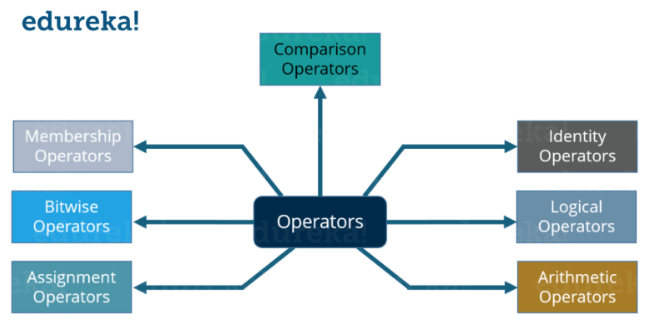Let’s focus on each of these Operators one by one

### Arithmetic Operators:

These Operators are used to perform mathematical operations like addition, subtraction etc. Assume that A = 10 and B = 20 for the below table.Consider the example below:

``````a = 21
b = 10
c = 0

c = a + b
print ( c )

c = a - b
print ( c )

c = a * b
print ( c )

c = a / b
print ( c )

c = a % b
print ( c )
a = 2
b = 3
c = a**b
print ( c )
``````

Output = 31, 11, 210, 2.1, 1, 8

Now let’s see comparison Operators.

#### Comparison Operators:

These Operators compare the values on either sides of them and decide the relation among them. Assume A = 10 and B = 20.Consider the example below:

``````a = 21
b = 10
c = 0

if ( a == b ):
print ("a is equal to b")
else:
print ("a is not equal to b")

if ( a != b ):
print ("a is not equal to b")
else:
print ("a is equal to b")

if ( a < b ): print ("a is less than b") else: print ("a is not less than b") if ( a > b ):
print ("a is greater than b")
else:
print ("a is not greater than b")

a = 5
b = 20
if ( a <= b ): print ("a is either less than or equal to b") else: print ("a is neither less than nor equal to b") if ( a => b ):
print ("a is either greater than  or equal to b")
else:
print ("a is neither greater than  nor equal to b")
``````
``````Output = a is not equal to b
a is not equal to b
a is not less than b
a is greater than b
a is either less than or equal to b
b is either greater than or equal to b
``````

Now in the above example, I have used conditional statements (if, else). It basically means if the condition is true then execute the print statement, if not then execute the print statement inside else.

### Assignment Operators:

An Assignment Operator is the operator used to assign a new value to a variable. Assume A = 10 and B = 20 for the below table.Consider the example below:

``````a = 21
b = 10
c = 0

c = a + b
print ( c )

c += a
print ( c )

c *= a
print ( c )

c /= a
print ( c )

c  = 2
c %= a
print ( c )

c **= a
print ( c )
``````

Output = 31, 52, 1092, 52.0, 2, 2097152, 99864

### Bitwise Operators:

These operations directly manipulate bits. In all computers, numbers are represented with bits, a series of zeros and ones. In fact, pretty much everything in a computer is represented by bits. Consider the example shown below: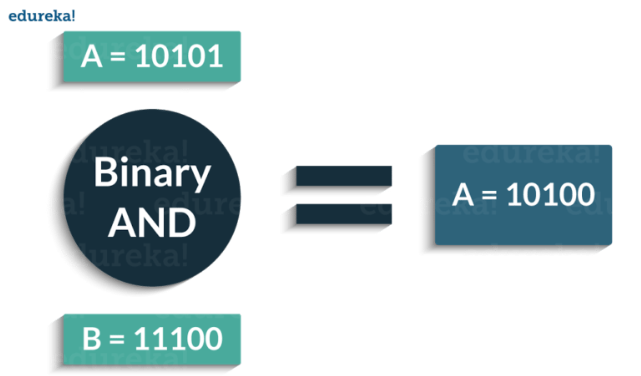Following are the Bitwise Operators supported by Python: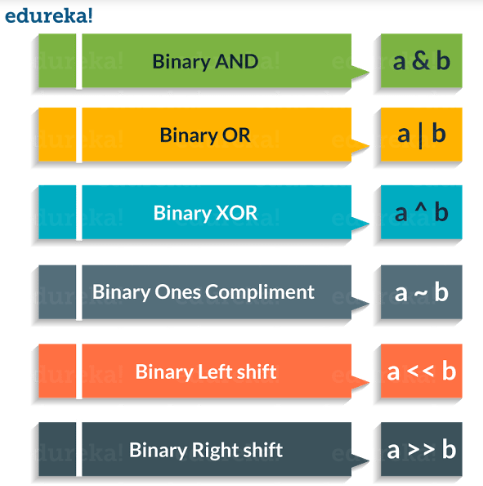Consider the example below:

``````a = 58        # 111010
b = 13        # 1101
c = 0

c = a & b
print ( c )   # 8 = 1000

c = a | b
print ( c )   # 63 = 111111

c = a ^ b
print ( c )   # 55 = 110111

c = a >> 2
print ( c )   # 232 = 11101000

c = a << 2
print ( c )   # 14 = 1110
``````

Output = 8,63,55,232,14

Next up, in this Python Tutorial we will focus on Logical Operators.

### Logical Operators: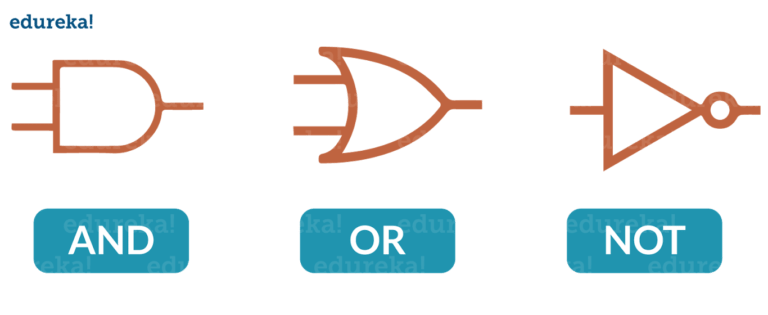The following are the Logical Operators present in Python:Consider the example below:

``````x = True
y = False

print('x and y is',x and y)

print('x or y is',x or y)

print('not x is',not x)
``````

Now in Python Tutorial, we’ll learn about Membership Operators.

### Membership Operators:

These Operators are used to test whether a value or a variable is found in a sequence (Lists, Tuples, Sets, Strings, Dictionaries) or not. The following are the Membership Operators:Consider the example below:

``````X = [1, 2, 3, 4]
A = 3
print(A in X)
print(A not in X)
``````
``````Output = True

False
``````

Next in Python Tutorial, it’s time we understand the last Operator i.e. Identity Operator.

### Identity Operators:

These Operators are used to check if two values (or variables) are located on the same part of the memory. Two variables that are equal does not imply that they are identical.

Following are the Identity Operators in Python:Consider the example below:

``````X1 = 'Welcome To edureka!'

X2 = 1234

Y1 = 'Welcome To edureka!'

Y2 = 1234

print(X1 is Y1)

print(X1 is not Y1)

print(X1 is not Y2)

print(X1 is X2)
``````
``````Output: True

False

True

False
``````

### Conditional Statements in Python

Consider the flowchart shown below: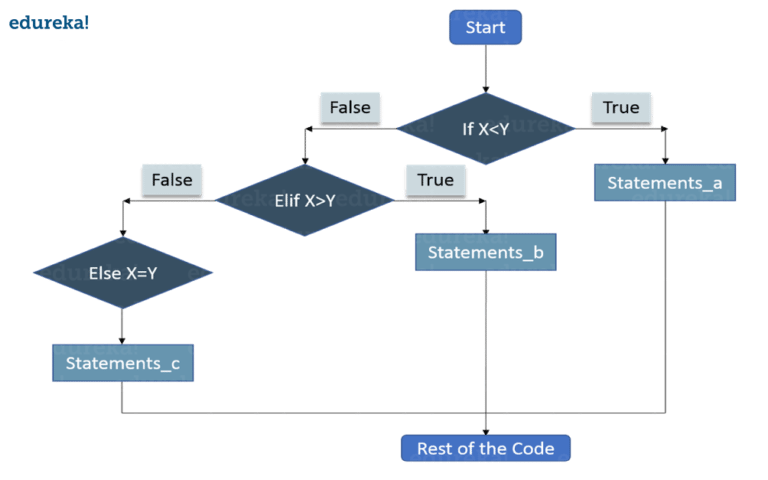Let me tell you how it actually works.

• ``````First the control will check the ‘If’ condition. If it is true, then the control will execute the statements after If condition.
``````
• ``````When ‘If’ condition is false, then the control will check the ‘Elif’ condition. If Elif condition is true then the control will execute the statements after Elif condition.
``````
• ``````If ‘Elif’ Condition is also false then the control will execute the Else statements.
``````

Syntax

``````if condition1:
statements

elif condition2:
statements

else:
statements
``````

Consider the example below:

``````X = 10
Y = 12

if X < Y: print('X is less than Y') elif X > Y:
print('X is greater than Y')
else:
print('X and Y are equal')
``````

`Output: X is less than Y`

## Loops

In general, statements are executed sequentially. The first statement in a function is executed first, followed by the second, and so on

There may be a situation when you need to execute a block of code several number of times

A loop statement allows us to execute a statement or group of statements multiple times. The following diagram illustrates a loop statement: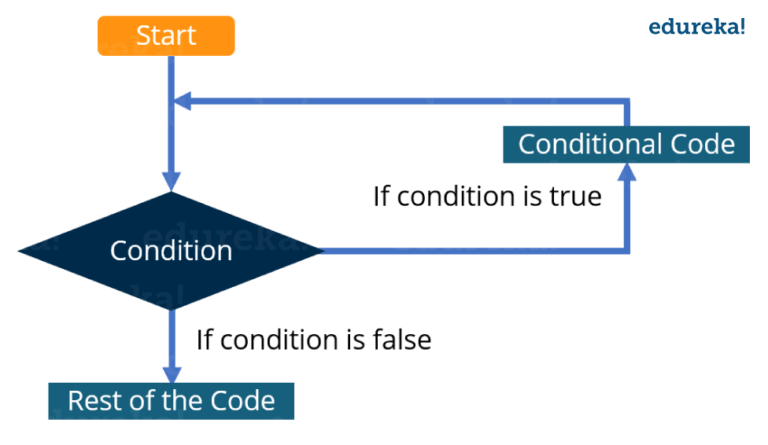Let me explain you the above diagram:

• ``````First the control will check the condition. If it is true then the control will move inside the loop and execute the statements inside the loop.
``````
• ``````Now, the control will again check the condition, if it is still true then again it will execute the statements inside the loop.
``````
• ``````This process will keep on repeating until the condition becomes false. Once the condition becomes false the control will move out of loop.
``````

There are two types of loops:

• ``````Infinite: When condition will never become false
``````
• ``````Finite: At one point, the condition will become false and the control will move out of the loop
``````

There is one more way to categorize loops:

• ``````Pre-test: In this type of loops the condition is first checked and then only the control moves inside the loop
``````
• ``````Post-test: Here first the statements inside the loops are executed, and then the condition is checked
``````

Python does not support Post-test loops.

### Loops in Python

In Python, there are three loops:

• ``````While
``````
• ``````For
``````
• ``````Nested
``````

#### While Loop

Here, first the condition is checked and if it’s true, control will move inside the loop and execute the statements inside the loop until the condition becomes false. We use this loop when we are not sure how many times we need to execute a group of statements or you can say that when we are unsure about the number of iterations.

Consider the example:

Syntax and Usage

``````count = 0
while (count < 10):
print ( count )
count = count + 1

print ("Good bye!")
``````
``````Output = 0
1
2
3
4
5
6
7
8
9
Good bye!
``````

#### For Loop

Like the While loop, the For loop also allows a code block to be repeated certain number of times. The difference is, in For loop we know the amount of iterations required unlike While loop, where iterations depends on the condition. You will get a better idea about the difference between the two by looking at the syntax:

Syntax

``````for variable in Sequence:
statements
``````

Notice here, we have specified the range, that means we know the number of times the code block will be executed.

Consider the example:

``````fruits = ['Banana', 'Apple',  'Grapes']

for index in range(len(fruits)):
print (fruits[index])
``````
``````Output: Banana        Apple         Grapes
``````

#### Nested Loops

It basically means a loop inside a loop. It can be a For loop inside a While loop and vice-versa. Even a For loop can be inside a For loop or a While loop inside a While loop.

Consider the example:

``````count = 1
for i in range(10):
print (str(i) * i)

for j in range(0, i):
count = count +1
``````
``````Output
1
22
333
4444
55555
666666
7777777
88888888
999999999
``````

Now is the best time to introduce functions in this Python Tutorial.

## File Input/Output In Python

Python has a built-in function open(), top open a file. This function returns a file object, also called a handle, as it is used to read or modify the file accordingly.

We can specify the mode while opening a file. In the mode, we specify whether we want to

• ``````read ‘r’
``````
• ``````write ‘w’ or
``````
• ``````append ‘a’ to the file. We also specify if we want to open the file in text mode or binary mode.
``````

The default is reading in text mode. In this mode, we get strings when reading from the file.

Syntax

``````o = open("edureka.txt")      # equivalent to 'r' or 'rt'
o = open ("edureka.txt",'w') # write in text mode
o = open ("img1.bmp",'rb' )  # read and write in binary mode
``````

### How To Close A File Using Python?

When we are done with operations to the file, we need to properly close the file.

Closing a file will free up the resources that were tied with the file and is done using Python close() method.

``````o = open ("edureka.txt")
o.close()
``````

## Python Modules

Modules are simply a ‘program logic’ or a ‘python script’ that can be used for variety of applications or functions. We can declare functions, classes etc in a module.

The focus is to break down the code into different modules so that there will be no or minimum dependencies on one another. Using modules in a code helps to write lesser line of codes, a single procedure developed for reuse of the code as well. It also eliminates the need to write the same logic again and again.

### Python Built-in Modules

Built-in modules are written in C and integrated with python interpreter. Each built-in module contains resources for certain specific functionalities like Operating system management, disk input/output etc.

The standard library also has many python scripts containing useful utilities. There are several built-in modules in python at our disposal that we can use whenever we want.

Some Of the Most Common and most useful built-in modules are:

• ``````OS
``````
• ``````MATH
``````
• ``````RANDOM
``````
• ``````DATETIME
``````

### OS Module In Python

The OS module in Python is a part of the standard library of the programming language. When imported, it lets the user interact with the native OS Python is currently running on. In simple terms, it provides an easy way for the user to interact with several os functions that come in handy in day to day programming.

Example: os.popen(): This function is a part of file object manipulation and is used to open a pipe to and from a command. The return value of this function can be read or written dependent upon your use of r or w. The syntax for this function is as follows, os.popen(command[, mode[, bufsize]]). The parameters taken into consideration are, mode as well as bufsize. Take a look at the example below to understand this better.

``````import os
fd = "GFG.txt"

# popen() is similar to open()
file = open(fd, 'w')
file.write("Hello")
file.close()
file = open(fd, 'r')
print(text)

# popen() provides a pipe/gateway and accesses the file directly
file = os.popen(fd, 'w')
file.write("Hello")
# File not closed, shown in the next function.
``````

Output: `Hello`

### Math Module In Python

Some of the most important mathematical functions are defined in the math module in python, the following example shows how we can use it in a program.

``````import math
print(math.pi)
#it will print the value of pi
``````

### Random Module In Python

Functions in the random module generate a pseudo-random number using the random() function that generates that generates a float number from 0.0 to 1.0.

The following example shows how we can use random in a program in python.

``````import random
print(random.random())
``````

### Datetime Module In Python

A date in python is not a type of its own, we can work on it by using the datetime module in python. The following example shows how we can use it in a program in python.

``````import datetime
print(datetime.datetime.now())
#it will print the current date and time
``````

## Python OOPs

OOPs refers to the Object-Oriented Programming in Python. Well, Python is not completely object-oriented as it contains some procedural functions. Now, you must be wondering what is the difference between a procedural and object-oriented programming. To clear your doubt, in a procedural programming, the entire code is written into one long procedure even though it might contain functions and subroutines. It is not manageable as both data and logic get mixed together. But when we talk about object-oriented programming, the program is split into self-contained objects or several mini-programs. Each object is representing a different part of the application which has its own data and logic to communicate among themselves. For example, a website has different objects such as images, videos etc.

### Python Classes

A class in python is the blueprint from which specific objects are created. It lets you structure your software in a particular way. Here comes a question how? Classes allow us to logically group our data and function in a way that it is easy to reuse and a way to build upon if need to be.

Using a class, you can add consistency to your programs so that they can be used in cleaner and efficient ways. The attributes are data members (class variables and instance variables) and methods which are accessed via dot notation.

• ``````**Class variable** is a variable that is shared by all the different objects/instances of a class.
``````
• ``````**Instance variables** are variables which are unique to each instance. It is defined inside a method and belongs only to the current instance of a class.
``````
• ``````**Methods** are also called as functions which are defined in a class and describes the behaviour of an object.
``````

To create a class in python, consider the below example:

``````class employee:
pass
#no attributes and methods
emp_1=employee()
emp_2=employee()
#instance variable can be created manually
emp_1.first='aayushi'
emp_1.last='Johari'
emp_1.email='aayushi@edureka.co'
emp_1.pay=10000

emp_2.first='test'
emp_2.last='abc'
emp_2.email='test@company.com'
emp_2.pay=10000
print(emp_1.email)
print(emp_2.email)
``````

Output:

`aayushi@edureka.co`
`test@company.com`

#### Python Inheritance

Inheritance allows us to inherit attributes and methods from the base/parent class. This is useful as we can create sub-classes and get all of the functionality from our parent class. Then we can overwrite and add new functionalities without affecting the parent class.

A class which inherits the properties is known as Child Class whereas a class whose properties are inherited is known as Parent class.

Say, I want to create classes for the types of employees. I’ll create ‘developers’ and ‘managers’ as sub-classes since both developers and managers will have a name, email and salary and all these functionalities will be there in the employee class. So, instead of copying the code for the sub-classes, we can simply reuse the code by inheriting from the employee.

``````class employee:
num_employee=0
raise_amount=1.04
def __init__(self, first, last, sal):
self.first=first
self.last=last
self.sal=sal
self.email=first + '.' + last + '@company.com'
employee.num_employee+=1
def fullname (self):
return '{} {}'.format(self.first, self.last)
def apply_raise (self):
self.sal=int(self.sal * raise_amount)
class developer(employee):
pass

emp_1=developer('aayushi', 'johari', 1000000)
print(emp_1.email)
``````

Output:

`aayushi.johari@company.com`

This brings us to the end of this article where we have learned Python programming. I hope you are clear with all that has been shared with you in this tutorial.

#python #web-development #machine-learning

## Buddha Community1574340419

## Guide to Python Programming Language

Description
The course will lead you from beginning level to advance in Python Programming Language. You do not need any prior knowledge on Python or any programming language or even programming to join the course and become an expert on the topic.

The course is begin continuously developing by adding lectures regularly.

Please see the Promo and free sample video to get to know more.

Hope you will enjoy it.

Basic knowledge
An Enthusiast Mind
A Computer
Basic Knowledge To Use Computer
Internet Connection
What will you learn
Will Be Expert On Python Programming Language
Build Application On Python Programming Language

#uide to Python #Guide to Python Programming #Guide to Python Programming Language #Python Programming #Python Programming Language1624291780

## Learn Python - Full Course for Beginners [Tutorial]

This course will give you a full introduction into all of the core concepts in python. Follow along with the videos and you’ll be a python programmer in no time!
⭐️ Contents ⭐
⌨️ (0:00) Introduction
⌨️ (1:45) Installing Python & PyCharm
⌨️ (6:40) Setup & Hello World
⌨️ (10:23) Drawing a Shape
⌨️ (15:06) Variables & Data Types
⌨️ (27:03) Working With Strings
⌨️ (38:18) Working With Numbers
⌨️ (48:26) Getting Input From Users
⌨️ (52:37) Building a Basic Calculator
⌨️ (1:03:10) Lists
⌨️ (1:10:44) List Functions
⌨️ (1:18:57) Tuples
⌨️ (1:24:15) Functions
⌨️ (1:34:11) Return Statement
⌨️ (1:40:06) If Statements
⌨️ (1:54:07) If Statements & Comparisons
⌨️ (2:00:37) Building a better Calculator
⌨️ (2:07:17) Dictionaries
⌨️ (2:14:13) While Loop
⌨️ (2:20:21) Building a Guessing Game
⌨️ (2:32:44) For Loops
⌨️ (2:41:20) Exponent Function
⌨️ (2:47:13) 2D Lists & Nested Loops
⌨️ (2:52:41) Building a Translator
⌨️ (3:04:17) Try / Except
⌨️ (3:21:26) Writing to Files
⌨️ (3:28:13) Modules & Pip
⌨️ (3:43:56) Classes & Objects
⌨️ (3:57:37) Building a Multiple Choice Quiz
⌨️ (4:08:28) Object Functions
⌨️ (4:12:37) Inheritance
⌨️ (4:20:43) Python Interpreter
📺 The video in this post was made by freeCodeCamp.org
The origin of the article: https://www.youtube.com/watch?v=rfscVS0vtbw&list=PLWKjhJtqVAblfum5WiQblKPwIbqYXkDoC&index=3

🔥 If you’re a beginner. I believe the article below will be useful to you ☞ What You Should Know Before Investing in Cryptocurrency - For Beginner
⭐ ⭐ ⭐The project is of interest to the community. Join to Get free ‘GEEK coin’ (GEEKCASH coin)!
Thanks for visiting and watching! Please don’t forget to leave a like, comment and share!

#python #learn python #learn python for beginners #learn python - full course for beginners [tutorial] #python programmer #concepts in python1624930726

## Automating WhatsApp Web with Alright and Python

Alright is a python wrapper that helps you automate WhatsApp web using python, giving you the capability to send messages, images, video, and files to both saved and unsaved contacts without having to rescan the QR code every time.

### Why Alright?

I was looking for a way to control and automate WhatsApp web with Python; I came across some very nice libraries and wrappers implementations, including:

So I tried

pywhatkit, a well crafted to be used, but its implementations require you to open a new browser tab and scan QR code every time you send a message, no matter if it’s the same person, which was a deal-breaker for using it.

I then tried

pywhatsapp,`which is based on`yowsupand require you to do some registration with`yowsup`before using it of which after a bit of googling, I got scared of having my number blocked. So I went for the next option.

I then went for WebWhatsapp-Wrapper. It has some good documentation and recent commits so I had hoped it is going to work. But It didn’t for me, and after having a couple of errors, I abandoned it to look for the next alternative.

PyWhatsapp by shauryauppal, which was more of a CLI tool than a wrapper, surprisingly worked. Its approach allows you to dynamically send WhatsApp messages to unsaved contacts without rescanning QR-code every time.

So what I did is refactoring the implementation of that tool to be more of a wrapper to easily allow people to run different scripts on top of it. Instead of just using it as a tool, I then thought of sharing the codebase with people who might struggle to do this as I did.

#python #python-programming #python-tutorials #python-programming-lists #selenium #python-dev-tips #python-developers #programming #web-monetization1602968400

## Python Tricks Every Developer Should Know

Python is awesome, it’s one of the easiest languages with simple and intuitive syntax but wait, have you ever thought that there might ways to write your python code simpler?

In this tutorial, you’re going to learn a variety of Python tricks that you can use to write your Python code in a more readable and efficient way like a pro.

### Let’s get started

Swapping value in Python

Instead of creating a temporary variable to hold the value of the one while swapping, you can do this instead

``````>>> FirstName = "kalebu"
>>> LastName = "Jordan"
>>> FirstName, LastName = LastName, FirstName
>>> print(FirstName, LastName)
('Jordan', 'kalebu')
``````

#python #python-programming #python3 #python-tutorials #learn-python #python-tips #python-skills #python-development1602666000

## How to Remove all Duplicate Files on your Drive via Python

Today you’re going to learn how to use Python programming in a way that can ultimately save a lot of space on your drive by removing all the duplicates.

### Intro

In many situations you may find yourself having duplicates files on your disk and but when it comes to tracking and checking them manually it can tedious.

Heres a solution

But How do we do it?

If we were to read the whole file and then compare it to the rest of the files recursively through the given directory it will take a very long time, then how do we do it?

The answer is hashing, with hashing can generate a given string of letters and numbers which act as the identity of a given file and if we find any other file with the same identity we gonna delete it.There’s a variety of hashing algorithms out there such as

• md5
• sha1
• sha224, sha256, sha384 and sha512

#python-programming #python-tutorials #learn-python #python-project #python3 #python #python-skills #python-tips